# Scale Drawings

GCSELevel 4-5AQACambridge iGCSEEdexcelEdexcel iGCSEOCRWJEC

## Scale Drawings

A scale drawing portrays a real object with the lengths in proportion to the lengths of the actual object, but reduced or enlarged by a scale factor.

You may be asked to find the scale (or scale factor), and/or calculate actual distances based on a scale drawing.

Level 4-5GCSEAQAEdexcelOCRWJECCambridge iGCSEEdexcel iGCSE

## Scales and Scale Factors

Below is a scale drawing of a tennis court with a scale $1\text{ cm}:4\text{ m}$ or $1:400$.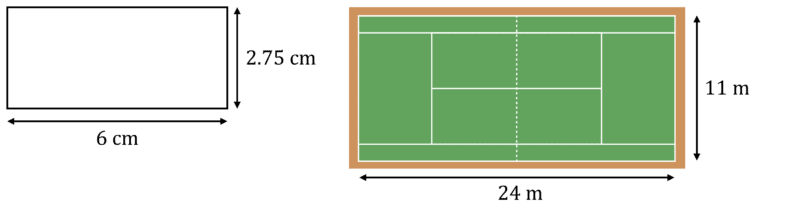We can use a scale of $1:400$ to calculate the scale factor, by dividing the left hand side of the ratio by the right hand side:

Scale factor: $1\div400=\dfrac{1}{400}$

This means, the actual tennis court size has been enlarged by a scale factor of $\dfrac{1}{400}$ to give the scale drawing.

If we did not have the scale, we could work out both the scale and the scale factor from the drawing:

Scale: we can use any length from the scale drawing and actual object to calculate this, making sure to choose the equivalent side in both.

Length scale drawing : Length actual object

$6\text{ cm}:24\text{ m}\\$ $6\text{ cm}:2400\text{ cm}\\$ $1\text{ cm}: 400\text{ cm}\\$ $1:400$

Scale factor: again, choose equivalent lengths from the scale drawing and actual image.

Length scale drawing $\div$ Length actual drawing

$6\div2400\\$ $1\div400\\$ $\dfrac{1}{400}$

Level 4-5GCSEAQAEdexcelOCRWJECCambridge iGCSEEdexcel iGCSE

## Calculating Lengths

We can use a scale or scale factor to calculate lengths or distances when given only a scale drawing.

Here is a scale drawing of a rug with scale $1:15$, calculate the length and width of the actual rug.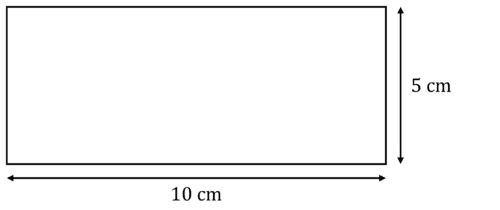Length: the length of the scale drawing is $10\text{ cm}$ and the scale is $1:15$.

The $1$ in this ratio represents the scale drawing, and the $15$ represents the actual rug, so the actual rug is $15$ times the size of the scale drawing.

Therefore, the length is $10\times15=150\text{ cm}$

Width: we can do the same here but using the value of the scale drawing’s width: $5\text{ cm}$.

Therefore, the width is $5\times15=75\text{ cm}$

Note: if we were told instead that the scale factor of the actual rug to the image is $\dfrac{1}{15}$ here, to find the length we would:

$10\div\dfrac{1}{15}=150\text{ cm}$

Level 4-5GCSEAQAEdexcelOCRWJECCambridge iGCSEEdexcel iGCSE

## Drawing Scale Diagrams

You may be asked to draw a scale drawing based off actual dimensions.

For example:

Below is an image of a rectangular door showing its actual dimensions. Given that the scale is $1:8$, draw a scale drawing of the door.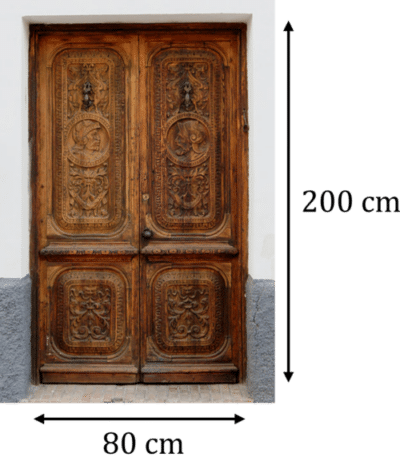To draw a scale drawing, you firstly need to work out the length of the sides of the drawing using the method we learnt previously:

As we have the actual object’s size, we will divide the width and height by $8$ to find the scaled lengths:

Width: $80\div8=10\text{ cm}$

Height: $200\div8=25\text{ cm}$

Therefore, we need to draw a rectangle with width $10\text{ cm}$, and height $25\text{ cm}$:Where the squares in the paper are $1\text{ cm}$

Level 4-5GCSEAQAEdexcelOCRWJECCambridge iGCSE
Level 4-5GCSEAQAEdexcelOCRWJECCambridge iGCSEEdexcel iGCSE

## Example 1: Scales and Scale Factors

Below is a scale drawing of a box in comparison to the actual box. Calculate the scale of this drawing.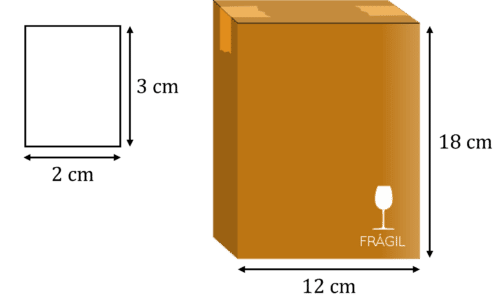[2 marks]

To calculate the scale, we need to find a ratio:

Length scale drawing : Length actual object

$2\text{ cm}:12\text{ cm}\\$ $1:6$

Note: we could have used the heights of this boxes to calculate this and it would have given the same answer.

Level 4-5GCSEAQAEdexcelOCRWJECCambridge iGCSEEdexcel iGCSE

## Example 2: Calculating Lengths

Below is a scale drawing of a ball. The actual ball has been enlarged by scale factor $\dfrac{1}{9}$. Calculate the diameter of the actual ball.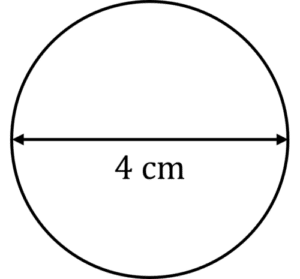[2 marks]

To calculate the diameter, we need to divide the scale drawing’s diameter by the scale factor:

$4\div\dfrac{1}{9}=36\text{cm}$

Level 4-5GCSEAQAEdexcelOCRWJECCambridge iGCSEEdexcel iGCSE

## Scale Drawings Example Questions

The scaled height is $3\text{ cm}$ and the scale is $1:5$, so we multiply $3$ by $5$ to find the actual height:

$3\times5=15\text{ cm}$Gold Standard Education

The ratio of scaled to actual is:

$12\text{ cm}:12\text{ m}$

Convert meters to centimeters:

$12\text{ cm}:1200\text{ cm}$

We can then divide this by $12$ to find the simplest form:

$1:100$Gold Standard Education

Firstly, multiply the scaled distance by the ratio:

$8\times120000=960000\text{ cm}$

To convert $\text{cm}$ to $\text{km}$, we divide by $100000$:

$960000\div100000=9.6\text{km}$Gold Standard EducationProduct